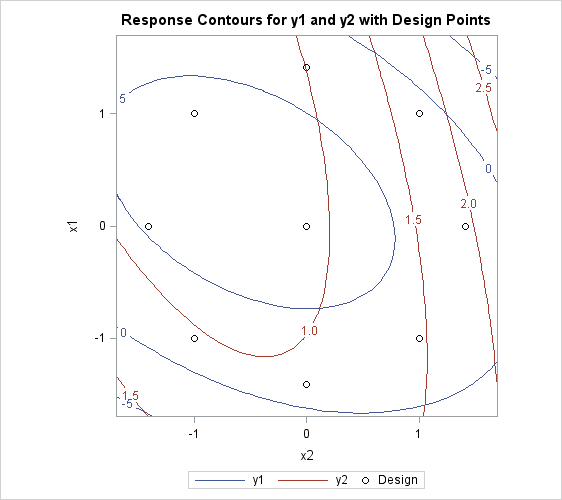Searching for Multiple Response Conditions

Suppose you have the following data with two factors and three responses, and you want to find the factor setting that produces responses in a certain region:

```data a;
input x1 x2 y1 y2 y3;
datalines;
-1      -1         1.8 1.940  3.6398
-1       1         2.6 1.843  4.9123
1      -1         5.4 1.063  6.0128
1       1         0.7 1.639  2.3629
0       0         8.5 0.134  9.0910
0       0         3.0 0.545  3.7349
0       0         9.8 0.453 10.4412
0       0         4.1 1.117  5.0042
0       0         4.8 1.690  6.6245
0       0         5.9 1.165  6.9420
0       0         7.3 1.013  8.7442
0       0         9.3 1.179 10.2762
1.4142  0         3.9 0.945  5.0245
-1.4142  0         1.7 0.333  2.4041
0       1.4142    3.0 1.869  5.2695
0      -1.4142    5.7 0.099  5.4346
;
```

You want to find the values of `x1` and `x2` that maximize `y1` subject to `y2`<2 and `y3`<`y2`+`y1`. The exact answer is not easy to obtain analytically, but you can obtain a practically feasible solution by checking conditions across a grid of values in the range of interest. First, append a grid of factor values to the observed data, with missing values for the responses:

```data b;
set a end=eof;
output;
if eof then do;
y1=.;
y2=.;
y3=.;
do x1=-2 to 2 by .1;
do x2=-2 to 2 by .1;
output;
end;
end;
end;
run;
```

Next, use PROC RSREG to fit a response surface model to the data and to compute predicted values for both the observed data and the grid, putting the predicted values in a data set `c`:

```proc rsreg data=b out=c;
model y1 y2 y3=x1 x2 / predict;
run;
```

Finally, find the subset of predicted values that satisfy the constraints, sort by the unconstrained variable, and display the top five predictions:

```data d;
set c;
if y2<2;
if y3<y2+y1;

proc sort data=d;
by descending y1;
run;

data d; set d;
if (_n_ <= 5);
proc print;
run;
```

The results are displayed in Figure 81.5. They indicate that optimal values of the factors are around 0.3 for `x1` and around –0.5 for `x2`.

Figure 81.5: Top Five Predictions

Obs x1 x2 _TYPE_ y1 y2 y3
1 0.3 -0.5 PREDICT 6.92570 0.75784 7.60471
2 0.3 -0.6 PREDICT 6.91424 0.74174 7.54194
3 0.3 -0.4 PREDICT 6.91003 0.77870 7.64341
4 0.4 -0.6 PREDICT 6.90769 0.73357 7.51836
5 0.4 -0.5 PREDICT 6.90540 0.75135 7.56883

If you are also interested in simultaneously optimizing `y1` and `y2`, you can specify the following statements to make a visual comparison of the two response surfaces by overlaying their contour plots:

```ods graphics on;
proc rsreg data=a plots=surface(overlaypairs);
model y1 y2=x1 x2;
run;
ods graphics off;
```

Figure 81.6 shows that you have to make some compromises in any attempt to maximize both `y1` and `y2`; however, you might be able to maximize `y1` while minimizing `y2`.

Figure 81.6: Overlaid Line Contours of Predicted Responses